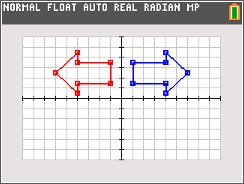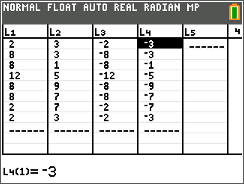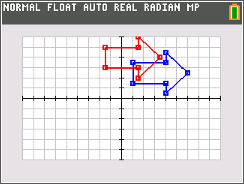# Activities

••• ##### Subject Area

• Math: Geometry: Transformational Geometry

• ##### Author9-12

45 Minutes

• ##### Device
• TI-84 Plus
• TI-84 Plus Silver Edition
•TI-84 Plus C Silver Edition
•TI-84 Plus CE

## Transformations With Lists#### Activity Overview

Students use list operations to perform reflections, rotations, translations and dilations on a figure, and graph the resulting image using a scatter plot.

#### Key Steps

•Students use the List Editor to enter values that gives the x- and y-values for a figure that is being rotated and reflected about the y-axis. They enter = –L1 and = –L2 in the formula bar of list 3 to create L3, the opposite of each of the x-values in L1. Then do a similar process for the y-values in L2.

•Students create a scatter plot with connected points using the combinations of lists. For each combination, students determine what type of reflection or rotation occurred.

•Students will translate a figure by manipulating formulas to translate the x- and y-values.